# FEA | FEA Model Updating

FEA Model Updating changes the Properties of selected FEA Objects on an FEA model so that its modal parameters more closely match a set of experimental (EMA) modal parameters.

To perform FEA Model Updating, the following items are required,

1. Mode shapes of the FEA structure corresponding to its current FEA properties

2. FEA structure model containing the FEA Objects with properties to be updated

3. EMA modal parameters

This command repeated executes the SDM command to solve for new mode shapes using FEA properties from a user-defined solution space of FEA Properties

• The FEA property solution space is defined by the Minimum, Maximum, and Number of Steps of each FEA property

• Solutions are ordered from best to worst

• The best solution is the one that minimizes the percent difference between the solution parameters and the EMA modal parameters

FEA | Point Matching is only required if MAC values between the FEA, Solution, and EMA mode shapes are desired.

## Difference Between SDM and FEA Model Updating

• SDM calculates the effects of additions or subtractions of FEA Objects to a structure model.

• FEA Model Updating calculates the effects of changes in the properties of existing FEA Objects on a structure model.

FEA Model Updating can be focused on portions of the structure to be updated, and FEA-EMA mode pairs to be used for ordering the solutions from best to worst. You can,

• Choose only the FEA Objects the properties of which are to be updated by hiding all other FEA Objects.

• Choose only the FEA Properties to be updated

• Choose only the pairs of FEA & EMA modal frequencies to be matched in the Error function used for ordering the solutions from best to worst

## Solution Order

The FEA Model Updating solutions are ordered from best to worst based on the values of an Error function.

• The best solution has FEA modal frequencies that are closest to selected EMA modal frequencies

• The Error function can also include the MAC values of the selected FEA-EMA mode pairs.

• If FEA-EMA mode pairs are not selected, the solutions are listed in the order that they are calculated.

## Command Requirements

Before this command can be executed, several items are required,

1. A structure model with FEA Objects attached to it

2. A Shape Table containing FEA mode shapes that display animate correctly of the FEA model.

3. A Shape Table containing EMA mode shapes

To initiate an FEA Model Updating calculation,

• Execute FEA | FEA Model Updating in the Structure window containing the FEA model to be updated

• The following dialog box will open.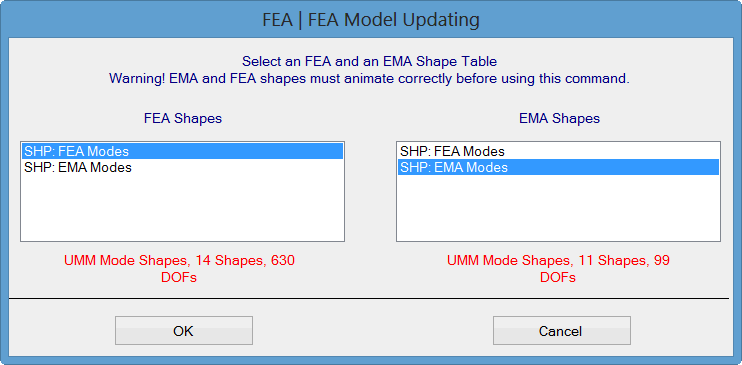Shape Table Selection Dialog Box.

• Choose a Shape Table containing the FEA Shapes on the left, and a Shape Table containing the EMA Shapes on the right

• Click on OK.

The FEA Model Updating window will open, as shown below.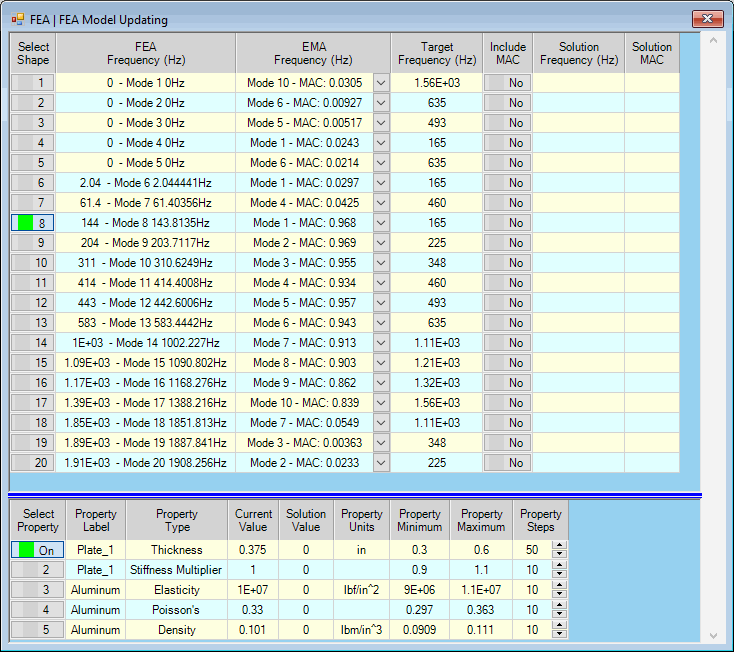FEA Model Updating Window Showing One Selected Mode Pair &One Selected Property for Updating.

The Upper spreadsheet list the modal frequencies and MAC values of all matching FEA-EMA mode pairs.  Each FEA-EMA mode pair is the pair with the maximum MAC value between all pairs of FEA and EMA mode shapes.

• The FEA modal frequencies are listed in the FEA Frequency (Hz) column

• The EMA modal frequencies for each matching FEA-EMA mode pair are listed in the EMA Frequency (Hz) column

• The MAC values for each matching FEA-EMA mode pair are also listed in the EMA Frequency (Hz) column

• The EMA modal frequencies of each matching FEA-EMA mode pair are listed in the Target Frequency (Hz) column

If FEA-EMA mode pairs are selected, only those pairs are used for calculating the Error function.  In none are selected, all pairs will be used for calculating the Error function.

The lower spreadsheet lists the FEA Properties of all visible FEA Objects on the structure model.

• The solution space for each Property is defined by its user-defined Minimum, Maximum, and Steps

• Minimum & Maximum define the lower & upper bounds of the Property solution space

• Steps is the number of steps to be evaluated between the Minimum & Maximum in the solution space

If properties are selected, only those properties are used to define the solution space.  In none are selected, all properties will be used to define the solution space.

## FEA Properties That Can Be Updated

Below is a Table of the Properties that can be updated using FEA Model Updating.

 FEA Object Property to Update Spring stiffness Mass mass Rod cross sectional area, material (Elasticity, Poisson's ratio, Density) Bar cross sectional area & inertias material (Elasticity, Poisson's ratio, Density) Plate thickness material (Elasticity, Poisson's ratio, Density) Solid material (Elasticity, Poisson's ratio, Density)

FEA Properties That Can Be Updated Using FEA Model Updating.

## Solution Space

Each Property to be used on the lower spreadsheet has a Minimum, Maximum & Steps column.  These three columns together define the solution space for the Property.

The Minimum, Maximum, & Steps of all (or selected) Properties in the lower spreadsheet define the solution space over which solutions are calculated.

• The Property Minimum & Maximum define the lower & upper bounds of the FEA Property in the solution space

• The Property Steps is the number of steps to be evaluated between the Minimum & Maximum Property values

• The total number of solutions is the product of the number of solutions of all (or selected) FEA Properties

### Solution Space Example

• If Property Minimum = 1, Property Maximum = 10 and Property Steps = 10, then 10 SDM solutions would be calculated for Property values (1,2,3,4,5,6,7,8,9,10)

• If three FEA Properties each have 10 Steps, then the solution space has a total of 1000 solutions.

## Calculation Process

• When Solution | Calculate is executed, FEA Model Updating solutions are calculated over the solution space of all (or selected) FEA Properties in the lower spreadsheet

• Exhaustive calculation using combinations of property values in the entire solution space guarantees that the best solution will always be found.

## Error Function

To order the solutions, an Error function is calculated for each solution.  The Error function is equal to the summation of the error between the frequencies of each selected FEA & EMA pair, as shown above.  If Include MAC is also depressed for a selected mode  pair, a second term (1 - MAC) is added to the Error function, as shown below

The use of mode shape MAC values is optional for calculating the Error function.

The formula for the Error function is: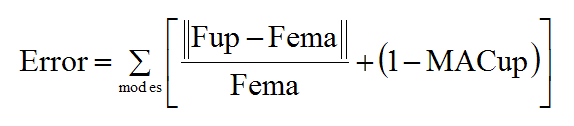where:

Fup = Updated FEA frequency

Fema = EMA frequency

MACup = MAC value between each Updated FEA & EMA mode shape pair.

As the solution calculation progresses through the solution space, the current minimum Error function is listed in the Error box on the Toolbar.

## Before Starting a Calculation

• Depress the Select Mode buttons to select each mode pair to be used in evaluating the Error function.

Only the modal parameters of the selected mode pairs will be used to calculate the Error function.

• Enter a Target frequency into the upper spreadsheet for each selected mode pair to be used for calculating the Error function.

If no Target modal parameters are entered for a selected mode pair, that pair will be excluded from the Error function calculation

• Depress the Select Property button in the lower spreadsheet to select each property to be used in the calculations

• Enter Property Minimum, Maximum & Steps into the lower spreadsheet to define the solution space for each FEA Property

If no properties are selected, the Minimum, Maximum & Step of all FEA Properties in the lower spreadsheet will be used to define the solution space.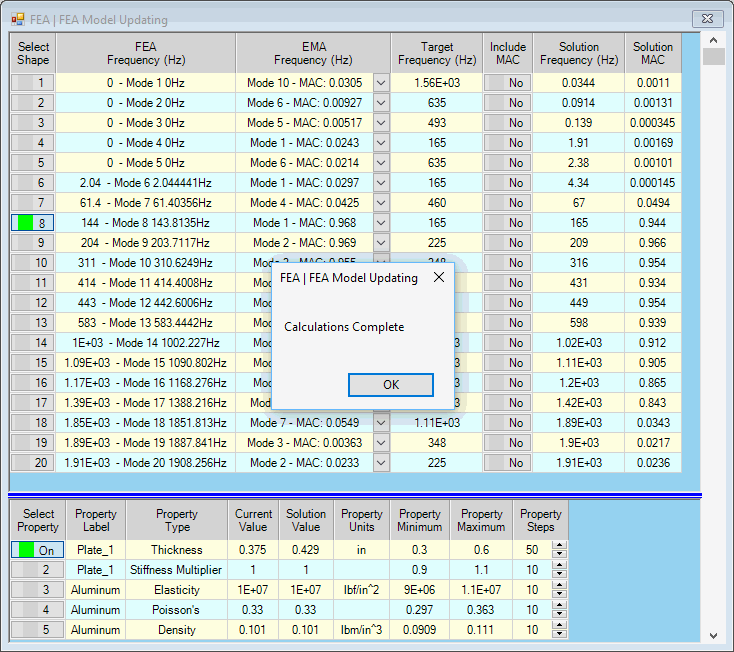FEA Model Updating Window After Solutions are Calculated.

## Solution | Calculate

Calculates the solutions for all (or selected) FEA Properties in the solution space defined in the lower spreadsheet.

• When all of the solutions have been calculated, the Solution Scroll Bar on the right side of the window will be enabled

## Solution | Stop Calculation

This command is enabled after Solution | Calculate has been executed.

• Execute Solution | Stop Calculation to stop the calculation process and display the solutions that have already been calculated.

## Solution Scroll Bar

After the calculation process is completed or stopped, the Solution scroll bar is enabled on the right hand side of the window.

• Parameters of the solutions are displayed in both the upper & lower spreadsheets by scrolling the Solution Scroll Bar

• If mode pairs are selected in the upper spreadsheet, the solutions are ordered according to their Error functions

• The best solution is displayed when the scroll is at the top of the Solution Scroll Bar

• The worst solution is displayed when the scroll is at the bottom of the Solution Scroll Bar

• If no mode pairs are selected in the upper spreadsheet, the solutions are ordered (from top to bottom of the Solution Scroll Barr) in the order in which they were calculated

## Display | Split

Toggles the spreadsheet display between Upper/Lower and Left/Right format.

When this command is checked, the solutions are displayed using two spreadsheets.

## Display | Bar Graphics

When this command is checked, the solutions are displayed graphically, as shown below.

• The upper left bar graph shows the percent difference between the Solution & Target frequency for each mode.

• Hover the mouse pointer over each bar on a bar graph to display its values.

• The upper right bar graph displays the MAC values between the Solution & FEA mode shapes.

• The lower bar graph displays the Current & Solution values of the Properties.

## Bar Charts

• Execute Display | Bar Charts to display the solutions using bar chart graphics.

The upper left bar graph shows two bars for each mode pair.

• The left (blue) bar is the Percent Difference between the FEA & EMA frequency.

• The right (green) bar is the Percent Difference between the Solution & Target frequency.

The upper right bar graph displays MAC values using two bars for each mode pair.

• The left (blue) bar is the MAC value between the FEA & EMA mode shapes.

• The right (red) bar is the MAC between the Solution & EMA mode shapes.

The lower bar graph displays the Current & Solution values of the FEA Properties.

• Hover the mouse pointer over each bar graph to display its numerical values.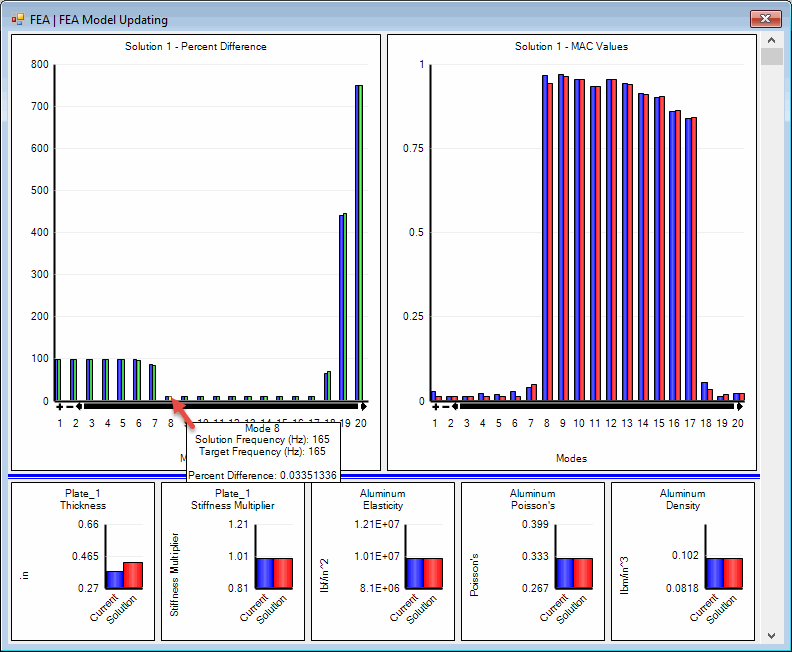Bar Chart Display.

## Solution | Save Solution Mode Shapes

Saves the mode shapes into a Shape Table that result from the current FEA Properties in the lower spreadsheet.

Before executing this command scroll the Solution Scroll Bar on the right hand side to display the desired solution.